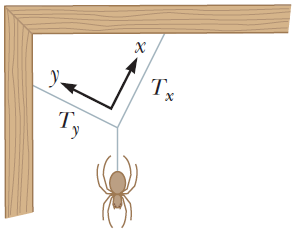# Problem: In the figure, a spider is resting after starting to spin its web. The gravitational force on the spider makes it exert a downward force of 0.150 N on the junction of the three strands of silk. The junction is supported by different tension forces in the two strands above it so that the resultant force on the junction is zero. The two sloping strands are perpendicular and we have chosen the x and y directions to be along them. The tension Tx is 0.127 N. Find (a) the tension Ty (b) the angle the x-axis makes with the horizontal and (c) the angle the y axis makes with the horizontal.

⚠️Our tutors found the solution shown to be helpful for the problem you're searching for. We don't have the exact solution yet.

###### Problem Details

In the figure, a spider is resting after starting to spin its web. The gravitational force on the spider makes it exert a downward force of 0.150 N on the junction of the three strands of silk. The junction is supported by different tension forces in the two strands above it so that the resultant force on the junction is zero. The two sloping strands are perpendicular and we have chosen the x and y directions to be along them. The tension Tx is 0.127 N. Find (a) the tension Ty (b) the angle the x-axis makes with the horizontal and (c) the angle the y axis makes with the horizontal.